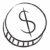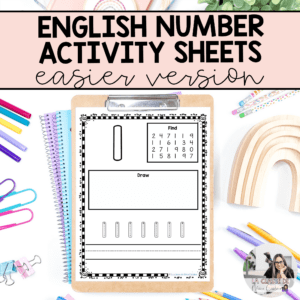0 Credits

# English Kindergarten Math Sheets: Numbers 1-20 (simpler version)

These activity sheets are helpful for teaching students how to write and represent numbers.

Skills Practiced:

• find the number
• trace the number
• write the number
• draw how many
• colour the number\$ 3.50

Credits

## Description

These activity sheets are helpful for teaching students how to write and represent numbers.

Skills Practiced:

• find the number
• trace the number
• write the number
• draw how many
• colour the number# English Kindergarten Math Sheets: Numbers 1-20 (simpler version)

\$ 3.50